Courses

# NCERT Solutions(Part- 1)- Playing with Numbers Class 8 Notes | EduRev

## Class 8 Mathematics by VP Classes

Created by: Full Circle

## Class 8 : NCERT Solutions(Part- 1)- Playing with Numbers Class 8 Notes | EduRev

The document NCERT Solutions(Part- 1)- Playing with Numbers Class 8 Notes | EduRev is a part of the Class 8 Course Class 8 Mathematics by VP Classes.
All you need of Class 8 at this link: Class 8

Question 1. Write the following numbers in generalized form.
(i) 25        (ii) 73
(iii) 129        (iv) 302

Solution:
(i) 25 = 20 + 5
= 10 x 2 + 5 x 1 = 10 x 2 + 5

(ii)  73 = 70 + 3
= 10 x 7 + 3 x 1 = 10 x 7 + 3

(iii) 129 = 100 + 20 + 9
= 100 x 1 + 10 x 2 + 1 x 9 = 100 x 1 + 10 x 2 + 9

(iv) 302 = 100 x 3 + 10 x 0 + 1 x 2 = 300 + 0 + 2

Question 2. Write the following in the usual form.
(i) 10 x 5 + 6     (ii) 100 x 7 + 10 x 1 + 8
(iii) 100 x a + 10 x c + b

Solution:
(i) 10 x 5 + 6 = 50 + 6 = 56
(ii) 100 x 7 + 10 x 1 + 8 = 700 + 10 + 8 = 718
(iii) 100 x a + 10 x c + b = 100a + 10c + b =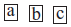[Adding the number with reversed digits to the chosen numbers.]
Question: Check what the result would have been if sundaram had chosen the numbers shown below.
1. 27
2. 39
3. 64
4. 17

Solution:
1.                Chosen number = 27
Number with reversed digits = 72
Sum of the two numbers = 27 + 72 = 99
Now,                                 99 = 11 = 11[2 + 7]
= 11[Sum of the digits of the chosen number]

2.                Chosen number = 39
Number with reversed digits = 93
Sum of the two numbers = 39 + 93 = 132
Now,                       132 ÷ 11 = 12
i.e.                                  132 = 11 = 11[3 + 9]
= 11[Sum of the digits of the chosen number]

3.                Chosen number = 64
Number with reversed digits = 46
Sum = 64 + 46 = 110
Now, 110 = 11 = 11[6 + 4]
= 11[Sum of the digits of the chosen number]

4.                Chosen number = 17
Number with reversed digits = 71
Sum = 17 + 71 = 88
Now,                                88 = 11 = 11[1+ 7]
= 11[Sum of the digits of the chosen number]

[Finding the difference of the chosen number and the number obtained by reversing the digits.]
Question: Check what result would have been if Sundaram had chosen the numbers shown.
1. 17
2. 21
3. 96
4. 37

Solution:
1.                Chosen number = 17
Number with reversed digits = 71
Difference = 71 – 17 = 54
= 9 x 
= 9 x [Difference of the digits of the chosen number (7 – 1 = 6)]

2.                Chosen number = 21
Number with reversed digits = 12
Difference = 21 – 12 = 9
= 9 x 
= 9 x [Difference between the digits of the chosen number (2 – 1 = 1)]

3.                 Chosen number = 96
Number with reversed digits = 69
Difference = 96 – 69 = 27
= 9 x 
= 9 x [Difference between the digits of the chosen number (9 – 6 = 3)]

4.                Chosen number = 37
Number with reversed digits = 73
Difference = 73 – 37 = 36
= 9 x 
= 9 x [Difference between the digits of the chosen number (7 – 3 = 4)]

Question: Check what the result would have been if Minakshi had chosen the numbers shown below.
In each case keep a record of the quotient obtained at the end.
1. 132
2. 469
3. 737
4. 901

Solution:
1.   132         Chosen number = 132
Reversed number = 231
Difference = 231 – 132 = 99
We have                 99 ÷ 99 = 1, remainder = 0

2.    469         Chosen number = 469
Reversed number = 964
Difference = 964 – 469 = 495
We have                 495 ÷ 99 = 5, remainder = 0

3.                 Chosen number = 737
Reversed number = 737
We have Difference = 737 – 737 = 0
0 ÷ 99 = 0, remainder = 0

4.               Chosen number = 901
Reversed number = 109
Difference = 901 – 109 = 792
We have           792 ÷ 99 = 8, remainder = 0
Forming three-digit number with given three digits

Note: Generalised form of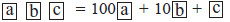Generalised form of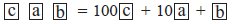Generalised form of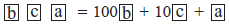Adding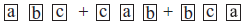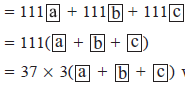which is divisible by 37

Question: Check what the result would have been if Sudaram had chosen the numbers shown below.
1. 417
2. 632
3. 117
4. 937

Solution:
1.          Chosen number = 417
Two other numbers with the same digits are 741 and 174
Sum of the three numbers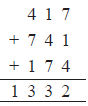We have 1332 ÷ 37 = 36, remainder = 0

2.            Chosen number = 632
Two other numbers are 263 and 326
Sum of the three numbers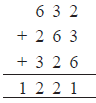We have 1221 ÷ 37 = 33, remainder = 0

3.        Chosen number = 117
Other numbers are 711 and 171
Sum of the three numbers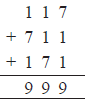We have 999 ÷ 37 = 27, remainder = 0

4.            Chosen number = 937
Other two numbers are 793 and 379
∴ Sum of the three numbers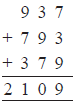We have 2109 ÷ 37 = 57, remainder = 0

EXERCISE 16.1
Question: Find the values of the letters in each of the following and give reasons for the steps
involved.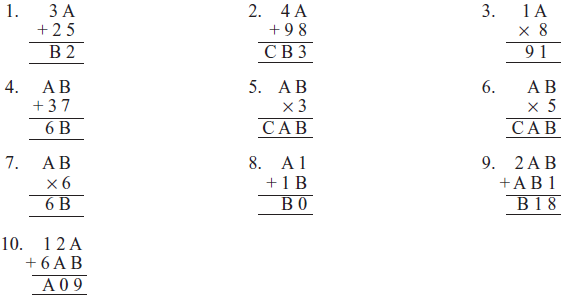Solution:
1. ∵                 A + 5 =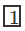2 or 22 or 32, etc.
∴        A = 12 – 5 = 7 or 22 – 5 = 17
Since                   A = 17 is not possible
∴                          A = 7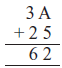Since+ 3 + 2 = 6
∴                          B = 6
Thus,                   A = 7, B = 6

2. If                   A + 8 =3
Then                    A = 13 – 8 = 5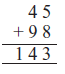∴+ 4 + 9 = B
or                           B =4
Clearly                   C = 1
Thus                      A = 5, B = 4 and C = 1

3. ∵           A x A = 1
∴                     A = 1, or A = 5 or A = 6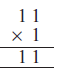If                    A = 1, then
∵                  11 ≠ 9A
∴                   A ≠ 1
If                  A = 5, then
75 ≠ 9A
∴               A = 5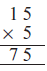If            A = 6, then
∵           96 = 9A
∴ A = 6 is the required values of A.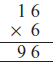4. We need B x 7 = A
∴ Possible value of B can be 5, [∵ 5 + 7 = 12]
∵ 6 – 3 – 1 = 2
∴ A can be equal to 2
Now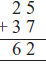∴ A = 2 and B = 5

5. We need B x 3 = 3B
Since 5 x 3 = 15
∴ Possible value of B can be 5.
Also         0 x 3 = 0, i.e. B = 0 can be another possible value
∵             A x 3 = A + 0 = A
∴ Possible value of A = 5 or A = 0
∴ Since C ≠ 0
∴ Possible value of A = 5
30, B must be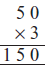Thus, = 0.
∴       A = 5, B = 0 and C = 1

6. B can be either 5 or 0.
∵                   A x 5 = A
∴ B must be 0
Again A can either be 5 or 0.
∴                  C ≠ 0    ∴ A ≠ 0
∴ A must be equal to 5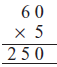Thus, we have
Therefore          A = 5, B = 0, C = 2

7. B can be 2, 4, 6 or 8
We need product 111 or 222, or 333 or 444 or 888 out of them 111 and 333 are rejected.
Possible products are 222, 444 or 888
To obtain
The possible value is B = 4
∵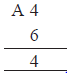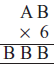∴      A can be either 2 or 7
A x 6 means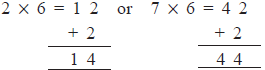∴                            A x 6 = 7 x 6 is the accepted value

Now,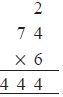Thus,           A = 7 and B= 4

8. ∵          10 – 1 = 9
∴                      B = 9
Also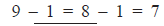∴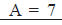We have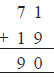Thus, A = 7 and B = 9

9. ∵             8 – 1 = 7
∴                       B = 7
∴                 7 + 4 = 11 ∴ A = 4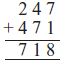Now, we have
Thus               A = 4 and B = 7

10. ∵             10 – 2 = 8
∴                          A = 8
Also               9 – 8 = 1 ∴ B = 1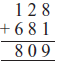Now, we have
Thus,                A = 8 and B = 1

Offer running on EduRev: Apply code STAYHOME200 to get INR 200 off on our premium plan EduRev Infinity!

93 docs|16 tests

,

,

,

,

,

,

,

,

,

,

,

,

,

,

,

,

,

,

,

,

,

;